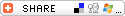#### Book

0 comments   /   posted by Marcel du Preez on Jun 15, 2010
Categories: Tutorials , Samples

### Introduction

Recently I have been working on a project, where a certain task's completion needs to be visually indicated in some graphic way. I settled on creating a style for the ProgressBar control, with a changing background color depending on the current value of the ProgressBar.

HSV (Hue-Saturation-Value)

From Wikipedia :

"HSL and HSV are the two most common cylindrical-coordinate representations of points in an RGB color model, which rearrange the geometry of RGB in an attempt to be more perceptually relevant than the cartesian representation."

HSV is a different way of representing a color (as opposed to RGB, where R indicates a value from 0 to 255 that "controls the amount of red", G for green and B for Blue). Have a look at the link above for an in-depth discussion.

I'll be using HSV to do my color change. By keeping the Saturation and Value values constant, and changing the Hue based on my progress, the color will change between Red, Orange, Yellow and finally Green.

IValueConverter

IValueConverter is an interface that can be implemented in WPF/Silverlight to provide a declarative way to convert properties from one datatype to another. I'll be using it to convert from my current progress (a int value between 0 and 100) and a Brush, which is what my ProgressBar expects as a background.

Create a class, and have it implement the IValueConverter interface, as below :

`01.``public` `class` `RedToGreenScaleConverter : IValueConverter `
`02.``{ `
`03.``    ``#region IValueConverter Members `
`04.`` `
`05.``    ``public` `object` `Convert(``object` `value, Type targetType, ``object` `parameter, System.Globalization.CultureInfo culture) `
`06.``    ``{ `
`07.``        ``return` `new` `SolidColorBrush(ColorFromHSV((``double``)value, 1, 1)); `
`08.``    ``} `
`09.`` `
`10.``    ``public` `object` `ConvertBack(``object` `value, Type targetType, ``object` `parameter, System.Globalization.CultureInfo culture) `
`11.``    ``{ `
`12.``        ``return` `null``; `
`13.``    ``} `
`14.``    ``#endregion `
`15.``}`

IValueConverter implements two methods : Convert, and ConvertBack. The titles should be self-explanatory. In ConvertBack, I return a null value (I don't want to convert a Brush to an int in my application). In Convert, I return a new SolidColorBrush, which I need to provide with one argument : a Color.

The Color is returned from a custom method call ColorFromHSV. It calculates the RGB values for specific H, S and V values (which are provided as arguments) :

`01.``private` `static` `Color ColorFromHSV(``double` `hue, ``double` `saturation, ``double` `value) `
`02.``        ``{ `
`03.``            ``int` `hi = (``int``)(Math.Floor(hue / 60)) % 6; `
`04.``            ``double` `f = hue / 60 - Math.Floor(hue / 60); `
`05.`` `
`06.``            ``value = value * 255; `
`07.``            ``int` `v = (``int``)(value); `
`08.``            ``int` `p = (``int``)(value * (1 - saturation)); `
`09.``            ``int` `q = (``int``)(value * (1 - f * saturation)); `
`10.``            ``int` `t = (``int``)(value * (1 - (1 - f) * saturation)); `
`11.`` `
`12.``            ``if` `(hi == 0) `
`13.``                ``return` `Color.FromArgb(255, (``byte``)v, (``byte``)t, (``byte``)p); `
`14.``            ``else` `if` `(hi == 1) `
`15.``                ``return` `Color.FromArgb(255, (``byte``)q, (``byte``)v, (``byte``)p); `
`16.``            ``else` `if` `(hi == 2) `
`17.``                ``return` `Color.FromArgb(255, (``byte``)p, (``byte``)v, (``byte``)t); `
`18.``            ``else` `if` `(hi == 3) `
`19.``                ``return` `Color.FromArgb(255, (``byte``)p, (``byte``)q, (``byte``)v); `
`20.``            ``else` `if` `(hi == 4) `
`21.``                ``return` `Color.FromArgb(255, (``byte``)t, (``byte``)p, (``byte``)v); `
`22.``            ``else`
`23.``                ``return` `Color.FromArgb(255, (``byte``)v, (``byte``)p, (``byte``)q); `
`24.``        ``}`

This code was adapted from here.

ProgressBar Style

To implement this in a ProgressBar, I needed to create a new style. Using Expression Blend, add a ProgressBar to your control/window/page, right-click on it in the Objects and Timeline window, select Edit Templates and Edit a Copy... :

This will generate a Style for a ProgressBar in the location you specify, based on the current style. Because I had no style specified, the default style is returned.

The style consists of a lot of XAML... but the part I'm interested in is called "Indicator", a rectangle. This rectangle contracts/expands depending on the Value property of the ProgressBar. I'll be changing it's Fill property, but first, a namespace declaration :

`1.``xmlns:converters="clr-namespace:ConverterDemo"`

and a reference to my converter in the Resources section

`1.``<``Window.Resources``> `
`2.``        ``<``converters:RedToGreenScaleConverter` `x:Key``=``"redGreenScale"` `/> `
`3.``</``Window.Resources``>`

and finally, changing the Fill property :

`1.``<``Rectangle` `x:Name``=``"Indicator"` `Fill``=``"{TemplateBinding Value, Converter={StaticResource redGreenScale}}"``/>`

This piece of code says that the Fill property should be set to the value of the ProgressBar's Value property (via TemplateBinding, check Bea Stollnitz's blog for a simple explanation), converted by using the RedToGreenScaleConverter. So, everytime the ProgressBar's value changes, that value will be converted to a Color by the converter.

### Result

This is what the ProgressBar looks like at the indicated values :Ciao!
Marcel du Preez
marcel@inivit.comRSS